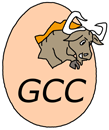# gfortran

Implementation of programming language Fortran

gfortran stands for GNU Fortran compiler, which is part of GCC — GNU Compiler Collection.

gfortran was created in January 2003 as a fork of an earlier project G95 (based on GCC back-end but not part of GCC). At that point G95 author was returning to single-person development mode, and collaborative efforts could be focused on gfortran instead. Since then the differences between the implementations grew significant enough to not be ignored.

gfortran is under active development now. At the moment it supports Fortran 95 fully and aims to provide compatibility with Fortran 2003 and Fortran 2008. gfortran developers recommend to use it instead of g77 since GCC 4.0 (the latter is not supported anymore).

### GNU Compiler Collection

GNU Compiler Collection (usually abbreviated as GCC) is a set of compilers for various programming languages developed within GNU project. GCC is free software and is distributed by Free Software Foundation (FSF) under GNU GPL and GNU LGPL licenses. It is used as a default compiler in free Unix-like operation systems as well as in some proprietary ones, including Apple Mac OS X.

All GCC compilers use a typical Unix interface: driver `gcc` interprets call arguments, chooses the compiler to be used, runs it, assembles and links the result to get the final output file.

Compilers for all languages use similar structure. The front-end parses program source code and builds an abstract syntax tree on it. The middle-end converts it to middle-level representation — either an architecture-independent GIMPLE or architecture-dependent RTL. Finally, the back-end generates a low-level program representation in machine codes.GCC logo

## Examples:

### Factorial:

Example for versions Intel Visual Fortran 11.1, gfortran 4.5.0

This example uses iterative factorial definition. Format specifiers I and A are used for printing integers and strings, respectively. Trying to calculate 13! produces an arithmetic overflow error, so last lines of program output look like this:

13! = 1932053504
14! = 1278945280
15! = 2004310016
16! = 2004189184

``````    program Factorial

integer :: f,n

f = 1
n = 0

do
print '(I2, A, I10)', n, "! = ", f
n = n + 1
f = f * n
if (n==17) then
exit
end if
end do

end program Factorial
``````

### Fibonacci numbers:

Example for versions Intel Visual Fortran 11.1, gfortran 4.5.0

This example uses iterative definition of Fibonacci numbers. The tricky part is printing the calculated values in one line, without leading or trailing spaces. Format specifier `(I3, A, \$)` means that first an integer is printed using exactly 3 positions, followed by a string. The final \$ suppresses trailing carriage return, used by default, so that everything is printed in one line.

``````    program Fibonacci

integer :: f1,f2,f3,i

i = 1
f1 = 0
f2 = 1

do
f3 = f2 + f1
f1 = f2
f2 = f3
i = i + 1
if (f1<10) then
print '(I1, A, \$)', f1, ', '
elseif (f1<100) then
print '(I2, A, \$)', f1, ', '
else
print '(I3, A, \$)', f1, ', '
end if
if (i==17) then
exit
end if
end do

print *, '...'

end program Fibonacci
``````

### Hello, World!:

Example for versions Intel Visual Fortran 11.1, gfortran 4.5.0
``````program HelloWorld

print *, 'Hello, World!'

end program HelloWorld
``````

Example for versions gfortran 4.5.0

This example uses built-in `complex` data type.

``````program Quadratic
integer a, b, c
real d, p1, p2
complex cp2

if (a.eq.0) then
write (*, *) "Not a quadratic equation"
stop
end if
d = b * b - 4 * a * c
p1 = - b / 2.0 / a
if (abs(d) < 1.0e-9) then
write (*, "(A, F8.3)") "x = ", p1
elseif (d > 0) then
p2 = sqrt(d) / 2.0 / a
write (*, "(2(A, F8.3))") "x1 = ", p1 + p2, char(13)//char(10)//"x2 = ", p1 - p2
else
cp2 = sqrt(cmplx(d)) / 2.0 / a
write (*, "(2(A, F8.3, F8.3), A)") "x1 = (", p1 + cp2, ")"//char(13)//char(10)//"x2 = (", p1 - cp2, ")"
end if
end
``````